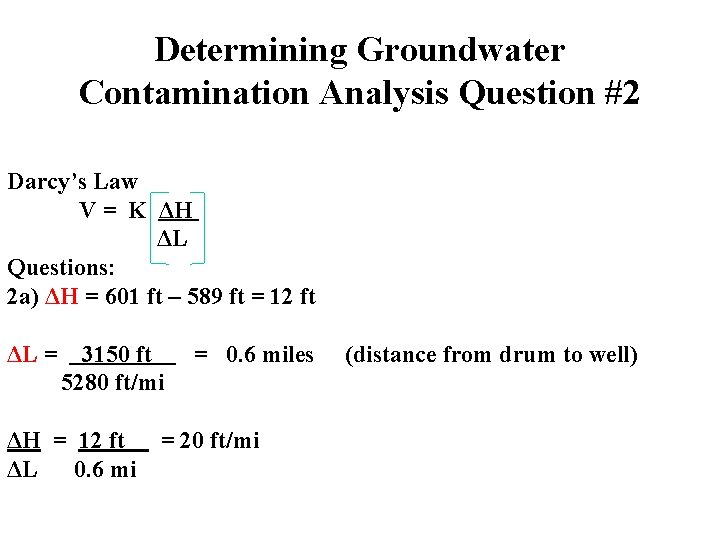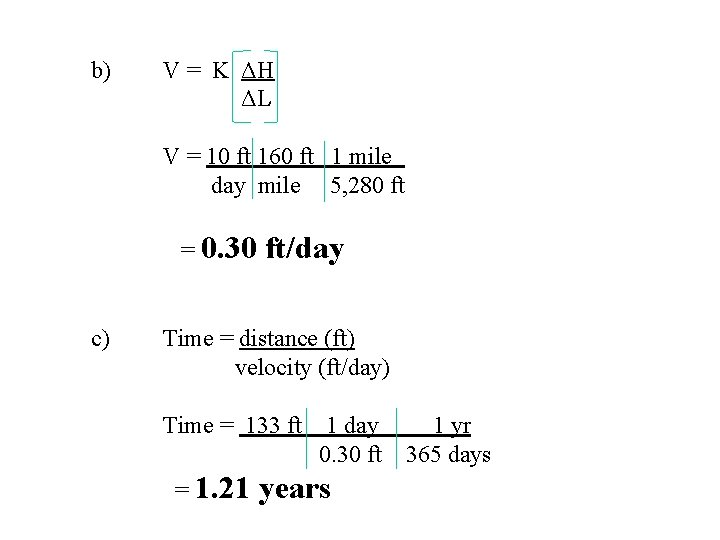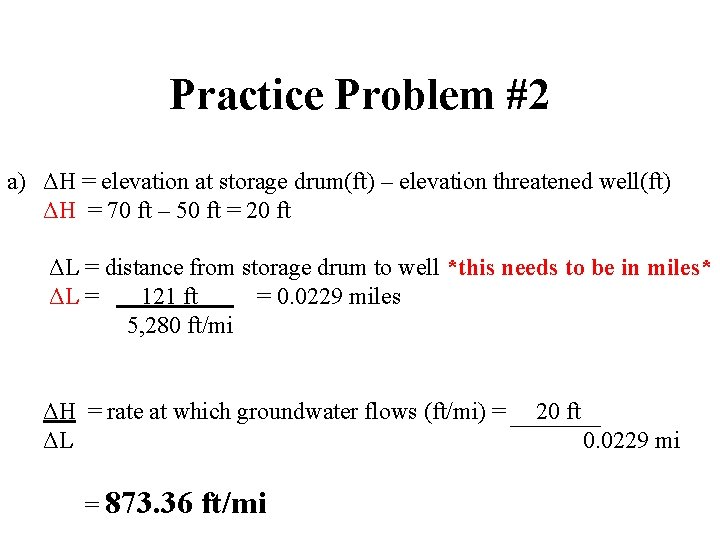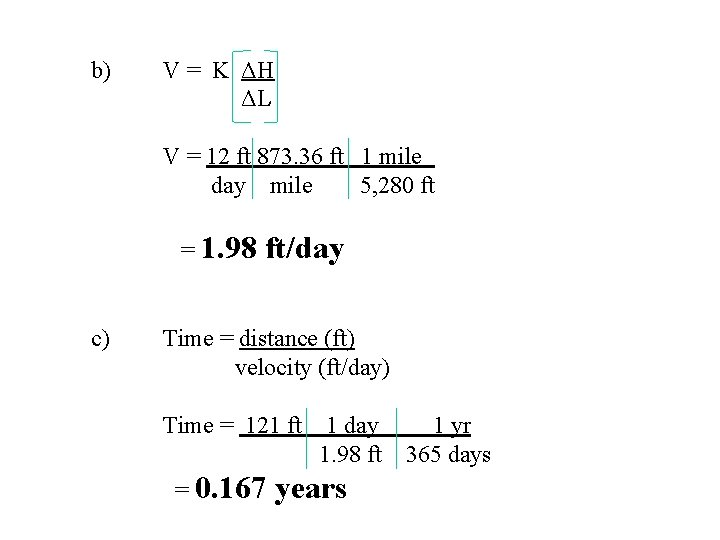# Determining Groundwater Contamination Determining Groundwater Contamination Darcys Law

• Slides: 9Determining Groundwater ContaminationDetermining Groundwater Contamination Darcy’s Law V = K ΔH ΔL Questions: 2 a) ΔH = elevation at storage drum(ft) – elevation threatened well(ft) ΔL = distance from storage drum to well (in miles) ΔH = rate at which groundwater flows (ft/mi) ΔL2 b) Velocity = K ΔH = ΔL Final answer in ft/day K= 100 ft/day 1 mile = 5280 ft 2 c) Time = distance = velocity Final answer in yearsDetermining Groundwater Contamination Analysis Question #2 Darcy’s Law V = K ΔH ΔL Questions: 2 a) ΔH = 601 ft – 589 ft = 12 ft ΔL = 3150 ft 5280 ft/mi = 0. 6 miles ΔH = 12 ft = 20 ft/mi ΔL 0. 6 mi (distance from drum to well)2 b) Velocity = K ΔH = (100 ft/day)(20 ft/mi)(1 mi/5, 280 ft) = 0. 4 ft/day ΔL K= 100 ft/day 1 mile = 5280 ft 2 c) Time = distance = (3, 150 ft)(1 day/0. 4 ft)(1 yr/365 days) = 21. 6 yrs velocityPractice Problem #1 a) ΔH = elevation at storage drum(ft) – elevation threatened well(ft) ΔH = 53 ft – 49 ft = 4 ft ΔL = distance from storage drum to well *this needs to be in miles* ΔL = 133 ft = 0. 025 miles 5, 280 ft/mi ΔH = rate at which groundwater flows (ft/mi) = ΔL = 160 ft/mi 4 ft 0. 025 mib) V = K ΔH ΔL V = 10 ft 160 ft 1 mile day mile 5, 280 ft = 0. 30 c) ft/day Time = distance (ft) velocity (ft/day) Time = 133 ft 1 day 0. 30 ft = 1. 21 years 1 yr 365 daysPractice Problem #2 a) ΔH = elevation at storage drum(ft) – elevation threatened well(ft) ΔH = 70 ft – 50 ft = 20 ft ΔL = distance from storage drum to well *this needs to be in miles* ΔL = 121 ft = 0. 0229 miles 5, 280 ft/mi ΔH = rate at which groundwater flows (ft/mi) = ΔL = 873. 36 ft/mi 20 ft 0. 0229 mib) V = K ΔH ΔL V = 12 ft 873. 36 ft 1 mile day mile 5, 280 ft = 1. 98 c) ft/day Time = distance (ft) velocity (ft/day) Time = 121 ft 1 day 1. 98 ft = 0. 167 years 1 yr 365 days